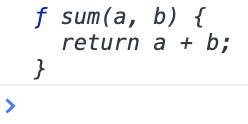Backbencher.dev

# JavaScript Basic Interview Questions

Last updated on 4 Nov, 2020

Question:

How are functions stored in memory by JavaScript? Here we have a function `sum()`.

``````function sum(a, b) {
return a + b;
}``````

How `sum()` function is stored in memory?

JavaScript allocates a memory block, just like a memory is allocated for a variable. The definition of the function is then stored inside the variable. That is why if we print the value of `sum` in console, it prints out the full source code of the function.

``console.log(sum);``

Here is the console output:----o----

Question:

JavaScript is single threaded. That means, it can execute one and only one part of the code at a time. If a code block calls a function, the block needs to wait until the function finishes its execution.

----o----

Question:

What is the difference between `undefined` and `null` in JavaScript?

`undefined` denotes a variable that is declared, but not yet initialized. In JavaScript, if we declare a variable and prints it value, the output will be `undefined`.

``````var a;
console.log(a); // undefined``````

On the other hand, `null` represents an intentional absense of an object. For example, the `match()` method in JavaScript returns all matches of a regular expression as an array.

``````var str = "some things are awesome";
var res = str.match(/some/g);
console.log(res); // ["some", "some"]``````

We can see that the `match()` method returns an array, which is an object. So we expect an object from `match()` method. But what if, there is no match? In that case, `null` is returned to denote intentional absense of an object.

``````var str = "some things are awesome";
var res = str.match(/boom/g);
console.log(res); // null``````